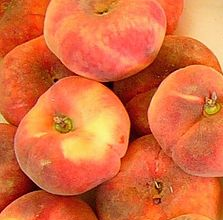#### You may also like### Tweedle Dum and Tweedle Dee

Two brothers were left some money, amounting to an exact number of pounds, to divide between them. DEE undertook the division. "But your heap is larger than mine!" cried DUM...### Sum Equals Product

The sum of the numbers 4 and 1 [1/3] is the same as the product of 4 and 1 [1/3]; that is to say 4 + 1 [1/3] = 4 ï¿½ 1 [1/3]. What other numbers have the sum equal to the product and can this be so for any whole numbers?### Special Sums and Products

Find some examples of pairs of numbers such that their sum is a factor of their product. eg. 4 + 12 = 16 and 4 × 12 = 48 and 16 is a factor of 48.

# Peaches Today, Peaches Tomorrow...

##### Age 11 to 14Challenge Level

This problem is in three parts. If you are feeling confident about working with fractions, you might want to skip straight to part (ii) or part (iii).(i) A little monkey had 60 peaches.

On the first day he decided to keep ${\bf \frac{3}{4}}$ of his peaches.
He gave the rest away. Then he ate one.

On the second day he decided to keep ${\bf \frac{7}{11}}$ of his peaches.
He gave the rest away. Then he ate one.

On the third day he decided to keep ${\bf \frac{5}{9}}$ of his peaches.
He gave the rest away. Then he ate one.

On the fourth day he decided to keep ${\bf \frac{2}{7}}$ of his peaches.
He gave the rest away. Then he ate one.

On the fifth day he decided to keep ${\bf \frac{2}{3}}$ of his peaches.
He gave the rest away. Then he ate one.

How many did he have left at the end?

(ii) A little monkey had 75 peaches.

Each day, he kept a fraction of his peaches, gave the rest away, and then ate one.
These are the fractions he decided to keep: $$\frac{1}{2} \qquad \frac{1}{4} \qquad \frac{3}{4} \qquad \frac{3}{5} \qquad \frac{5}{6} \qquad \frac{11}{15}$$
In which order did he use the fractions so that he was left with just one peach at the end?

(iii) Whenever the monkey has some peaches, he always keeps a fraction of them each day, gives the rest away, and then eats one.
I wonder how long he could make his peaches last for...
Here are his rules:
• Each fraction must be in its simplest form and must be less than 1.
• The denominator can never be the same as the number of peaches left.
For example, if there were 45 peaches left, he could not choose to keep $\frac{44}{45}$ of them.
Can you start with fewer than 100 peaches and choose fractions so that there is at least one peach left after a week?

Starting with fewer than 100, what is the longest you can make the peaches last?